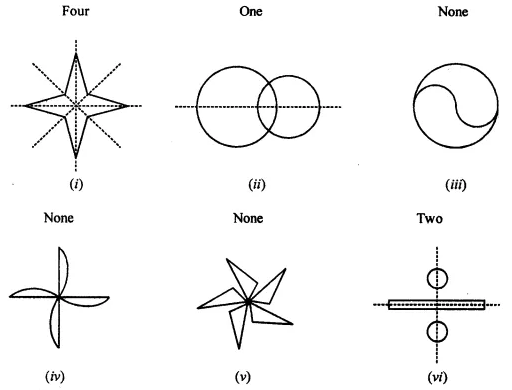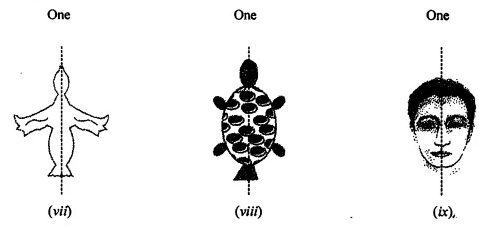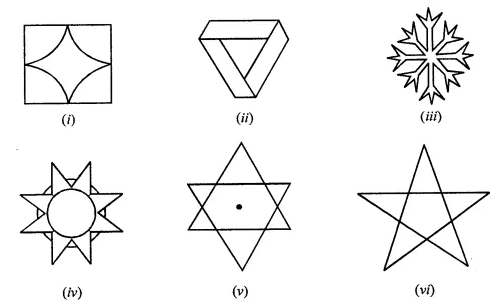# ML Aggarwal Class 7 Solutions for ICSE Maths Chapter 14 Symmetry Check Your Progress

## ML Aggarwal Class 7 Solutions for ICSE Maths Chapter 14 Symmetry Check Your Progress

Question 1.
Draw the line (or lines) of symmetry, if any of the following shapes and count their number.Solution:Question 2.
For each of the given shape in Question 1, find the order of the rotational symmetry (If any).
Solution:Question 3.
Give the order of rotational symmetry of each of the following figures:Solution: